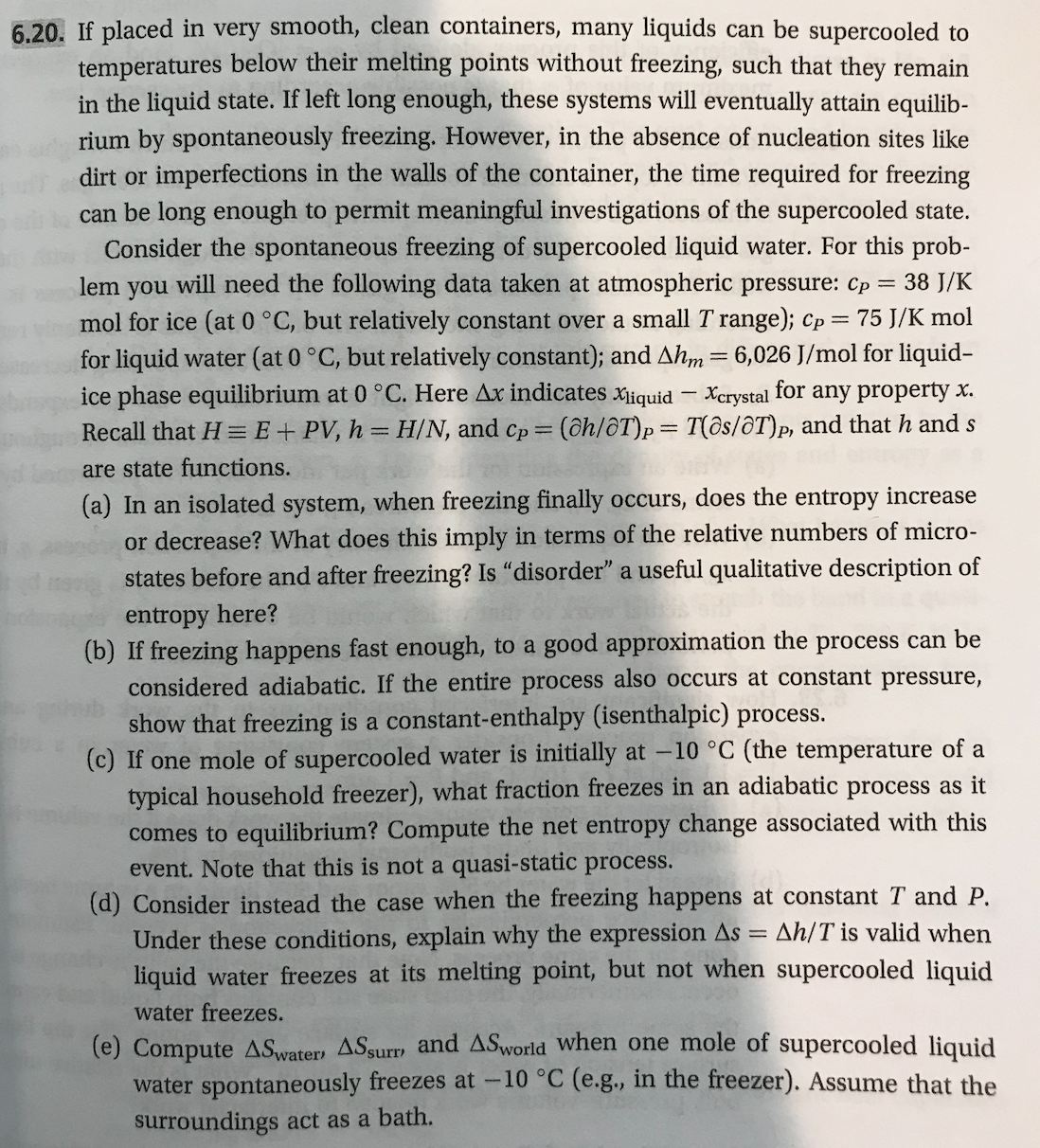6.20. If placed in very smooth, clean containers, many liquids can be supercooled totemperatures below their melting points without freezing, such that they remainin the liquid state. If left long enough, these systems will eventually attain equilib-rium by spontaneously freezing. However, in the absence of nucleation sites likedirt or imperfections in the walls of the container, the time required for freezingcan be long enough to permit meaningful investigations of the supercooled state.Consider the spontaneous freezing of supercooled liquid water. For this problem you will need the following data taken at atmospheric pressure: cp 38 J/Kmol for ice (at 0 °C, but relatively constant over a small T range); Cp-75 J/K molfor liquid water (at 0 °C, but relatively constant); and Δhm-6, 026 J/mol for liquid-ice phase equilibrium at 0 °C. Here Ax indicates iquid Xcrystal for any property xRecall that E + PV, h = H/N, and Cp = (ah/01),-7(os/OT)P, and that h and sare state functions(a) In an isolated system, when freezing finally occurs, does the entropy increaseor decrease? What does this imply in terms of the relative numbers of micro-states before and after freezing? Is "disorder" a useful qualitative description ofentropy here?(b) If freezing happens fast enough, to a good approximation the process can beconsidered adiabatic. If the entire process also occurs at constant pressure,how that freezing is a constant-enthalpy (isenthalpic) process(C) If one mole ofsupercooled water is initially at -10 °C (the temperature of atypical household freezer), what fraction freezes in an adiabatic process as itcomes to equilibrium? Compute the net entropy change associated with thisevent. Note that this is not a quasi-static process.(d) Consider instead the case when the freezing happens at constant T and P.Under these conditions, explain why the expression Δ:-Δh/T is valid whenliquid water freezes at its melting point, but not when supercooled liquidwater freezes.(e) Compute ASwater, ASsurw and ASworld when one mole of supercooled liquidater spontaneously freezes at -10 °C (e.g, in the freezer). Assume that thesurroundings act as a bath

Question

This problem is (6.20) from a book  "Thermodynamics and Statistical Mechanics An Integrated Approach by M. Scott Shell"help_outlineImage Transcriptionclose6.20. If placed in very smooth, clean containers, many liquids can be supercooled to temperatures below their melting points without freezing, such that they remain in the liquid state. If left long enough, these systems will eventually attain equilib- rium by spontaneously freezing. However, in the absence of nucleation sites like dirt or imperfections in the walls of the container, the time required for freezing can be long enough to permit meaningful investigations of the supercooled state. Consider the spontaneous freezing of supercooled liquid water. For this prob lem you will need the following data taken at atmospheric pressure: cp 38 J/K mol for ice (at 0 °C, but relatively constant over a small T range); Cp-75 J/K mol for liquid water (at 0 °C, but relatively constant); and Δhm-6, 026 J/mol for liquid- ice phase equilibrium at 0 °C. Here Ax indicates iquid Xcrystal for any property x Recall that E + PV, h = H/N, and Cp = (ah/01),-7(os/OT)P, and that h and s are state functions (a) In an isolated system, when freezing finally occurs, does the entropy increase or decrease? What does this imply in terms of the relative numbers of micro- states before and after freezing? Is "disorder" a useful qualitative description of entropy here? (b) If freezing happens fast enough, to a good approximation the process can be considered adiabatic. If the entire process also occurs at constant pressure, how that freezing is a constant-enthalpy (isenthalpic) process (C) If one mole of supercooled water is initially at -10 °C (the temperature of a typical household freezer), what fraction freezes in an adiabatic process as it comes to equilibrium? Compute the net entropy change associated with this event. Note that this is not a quasi-static process. (d) Consider instead the case when the freezing happens at constant T and P. Under these conditions, explain why the expression Δ:-Δh/T is valid when liquid water freezes at its melting point, but not when supercooled liquid water freezes. (e) Compute ASwater, ASsurw and ASworld when one mole of supercooled liquid ater spontaneously freezes at -10 °C (e.g, in the freezer). Assume that the surroundings act as a bath fullscreen
Step 1

Note: Since we only answer up to 3 sub-parts, we’ll answer the first 3. Please resubmit the question and specify the other subparts (up to 3) you’d like answered.

Part (a)

For an isolated system, dH = TdS. Thus,

Step 2

Freezing of supercooled water implies that, T ≤ 273.15 K.

Step 3

As T < 273.15 K, ΔStotal > 0, thus the process becomes irreversible. This happens when heat flows out of the system and freezing occurs, increasing the entropy of the system.

In an isolated system, ice is not formed at 0°C (273.15 ) but the liquid is supercooled liquid. For the formation of ice at this ...

Want to see the full answer?

See Solution

Want to see this answer and more?

Our solutions are written by experts, many with advanced degrees, and available 24/7

See Solution
Tagged in

Chemical Engineering